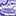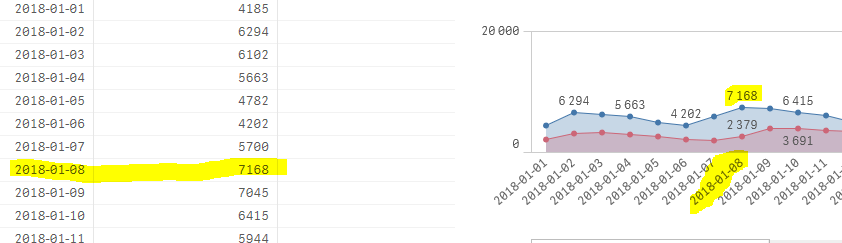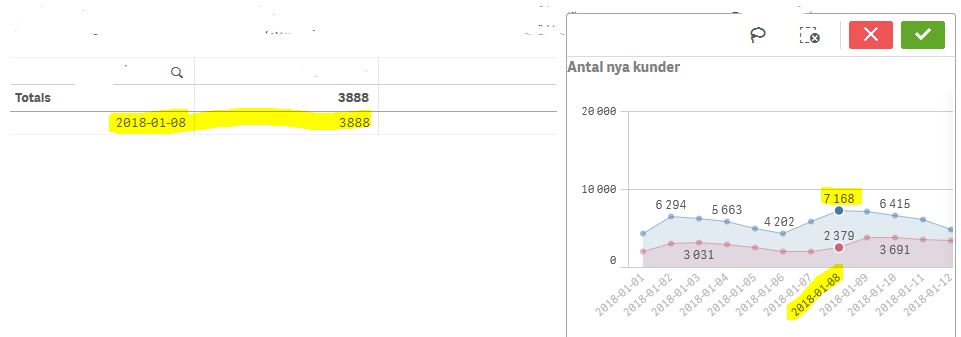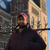# App Development

Announcements
cancel
Showing results for
Did you mean:Contributor III

## Before selecting: wrong data; After selecting: correct data

I will post 3 images, each having a table and a line chart.

The used data is exactly the same (it's using the same measure and the same dimensions).

PROBLEM:

1a. the data shown, before any selection, is wrong both in the table and in the line chart (see 1st image).

1b. After a selection is made (click on a point in the line chart - *without confirming* the selection), the data is correct in the table (see 2nd image).

1c. After a selection is made (click on a point in the line chart - *with confirming* the selection), the data is correct both in the table and in the line chart (see 3rd image).

QUESTION: why is the data displayed before any selection is wrong and after selection is correct? How to fix it?

1st image2nd image3rd image1 Solution

Accepted SolutionsContributor III
Author

The problem are the 2 variables vDateStart and vDateEnd. They are the MIN and MAX of Datum (not the "datum" used in the formula posted above). But the issue is that, for some reason, the variables do not get correctly calculated when they are used in the formula mentioned above:

 LET mNewCustomerCount = 'SUM(' & Chr(36) & '(setActuals) IF([datum] >= ' & Chr(36) & '(vDateStart) AND [datum] <= ' & Chr(36) & '(vDateEnd), c_distinct_count))';

Not sure how to fix the problem, but that is the problem. I used hardcoded dates in the formula, instead of using the variables ​vDateStart and vDateEnd, and the results were correct. It might be a Qlik Sense bug, not sure.

8 RepliesPartner

what is the expression that you are using?

I can walk on water when it freezesChampion

Hi,

would you be able to attach sample QVF file ?Contributor III
Author

Hey, thanks for the replay. The date is the measure and the dimension is:

 LET mNewCustomerCount = 'SUM(' & Chr(36) & '(setActuals) IF([datum] >= ' & Chr(36) & '(vDateStart) AND [datum] <= ' & Chr(36) & '(vDateEnd), c_distinct_count))';Contributor III
Author

wish I could, but no. thanks anywayPartner

why you are complicating things!

remove the if else condition and put it in the set analysis:

sum({<datum={">=\$(vDateStart) <=\$(vDateEnd)"}>}c_distinct_count)

what is in setActuals?

I can walk on water when it freezesContributor III
Author

setActuals = it's a flag for this year or last year.

But the behavior is strange, don't think the formula is wrong. Why does it show correct results when I specifically select a date, and wrong results when the dates are NOT selected....?Partner

I never use if else in expression

always try to put all login in set analysis format

I can walk on water when it freezesContributor III
Author

The problem are the 2 variables vDateStart and vDateEnd. They are the MIN and MAX of Datum (not the "datum" used in the formula posted above). But the issue is that, for some reason, the variables do not get correctly calculated when they are used in the formula mentioned above:

 LET mNewCustomerCount = 'SUM(' & Chr(36) & '(setActuals) IF([datum] >= ' & Chr(36) & '(vDateStart) AND [datum] <= ' & Chr(36) & '(vDateEnd), c_distinct_count))';

Not sure how to fix the problem, but that is the problem. I used hardcoded dates in the formula, instead of using the variables ​vDateStart and vDateEnd, and the results were correct. It might be a Qlik Sense bug, not sure.